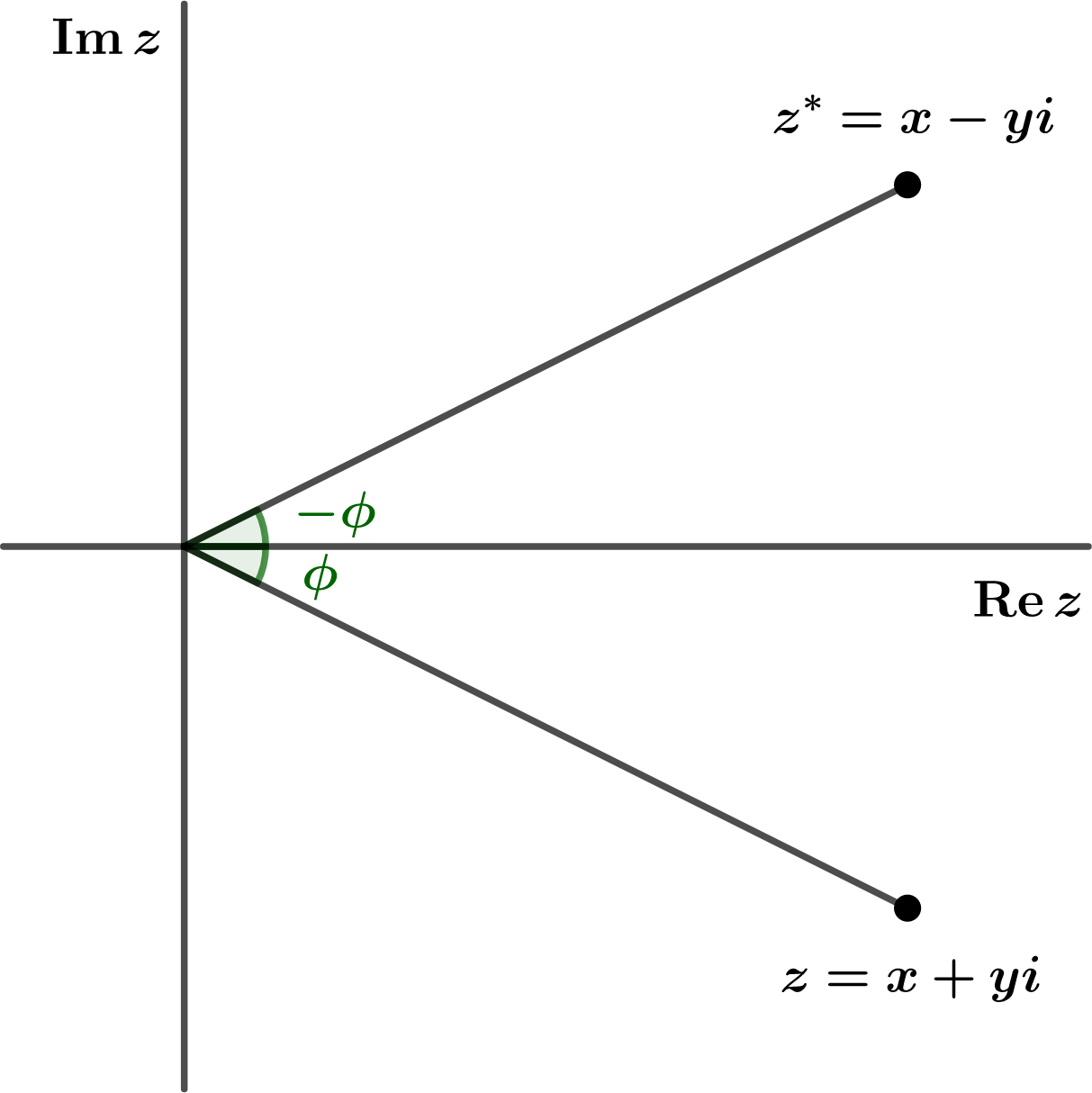The complex conjugate $$z^*$$ of a complex number $$z=x+iy$$ is found by replacing every $$i$$ by $$-i\text{.}$$ Therefore $$z^*=x-iy\text{.}$$ (A common alternate notation for $$z^*$$ is $$\bar{z}\text{.}$$) Geometrically, you should be able to see that the complex conjugate of ANY complex number is found by reflecting in the real axis, as shown in Figure 2.2.1.Figure 2.2.1. The point $$z=x+iy$$ and its complex conjugate $$z^*=x-iy\text{.}$$
Notice that this product is ALWAYS a positive, real number. The (positive) square root of this number is the distance of the point $$z$$ from the origin in the complex plane. We call the square root the norm or magnitude of $$z$$ and we use the same notation as "absolute value," i.e. $$\vert z\vert\text{.}$$ In this way, we see that the definition of absolute value, as in $$\vert -2\vert=2\text{,}$$ was never "strip off the minus sign," but really "how far is $$-2$$ from the origin."